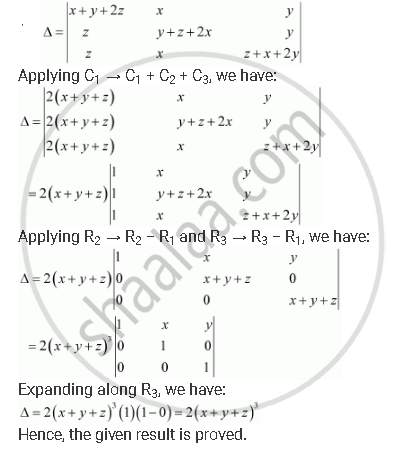Share

# By Using Properties of Determinants, Show That: |(X+Y+2z, X, Y),(Z, Y+Z+2z,Y),(Z,X,Z+X+2y)| = 2(X+Y+Z)Cuberoot3 - CBSE (Science) Class 12 - Mathematics

#### Question

By using properties of determinants, show that:

|(x+y+2z, x, y),(z, y+z+2z,y),(z,x,z+x+2y)| = 2(x+y+z)^3

#### SolutionIs there an error in this question or solution?

#### APPEARS IN

Solution By Using Properties of Determinants, Show That: |(X+Y+2z, X, Y),(Z, Y+Z+2z,Y),(Z,X,Z+X+2y)| = 2(X+Y+Z)Cuberoot3 Concept: Properties of Determinants.
S